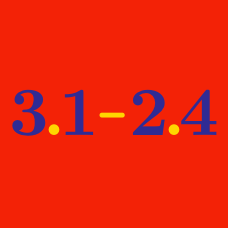Basic Mathematics

# Multiplying Decimals

What is $$0.25 \times 28$$?

What is the value of $$0. 3 \times 1.3$$?

What is the value of $$0. 4 \times 0.8$$?

What is $$0.8 \times 4 0$$?

What is the value of $$0. 2 \times 0.3$$?

×